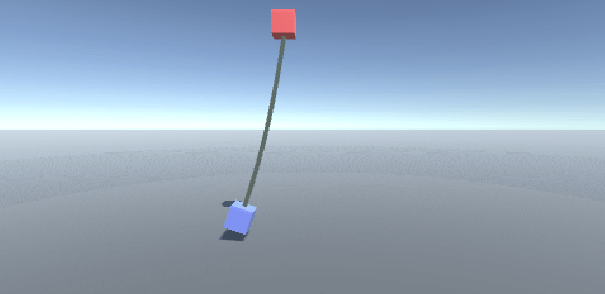# How to create a swinging rope in Unity

## 3. Another simplified rope

### Introduction

The problem with the realistic rope was that it was difficult to make it less bouncy and the problem with the second rope was that it can't swing around itself because it consists of just one long spring. A solution to this problem is to throw out the springs and use Verlet integration instead of an Euler method.

### Unity

To make this work you need two boxes. The rope will be attached to one of these boxes and the other box will simply hang from the rope. Then add the following script to a gameobject which has a line renderer attached to it:

```using System.Collections;
using System.Collections.Generic;
using UnityEngine;

//Simulate a rope with verlet integration and no springs
public class RopeControllerRealisticNoSpring : MonoBehaviour
{
//Objects that will interact with the rope
public Transform whatTheRopeIsConnectedTo;
public Transform whatIsHangingFromTheRope;

//Line renderer used to display the rope
private LineRenderer lineRenderer;

//A list with all rope section
private List<RopeSection> allRopeSections = new List<RopeSection>();

//Rope data
private float ropeSectionLength = 0.5f;

private void Start()
{
//Init the line renderer we use to display the rope
lineRenderer = GetComponent<LineRenderer>();

//Create the rope
Vector3 ropeSectionPos = whatTheRopeIsConnectedTo.position;

for (int i = 0; i < 15; i++)
{

ropeSectionPos.y -= ropeSectionLength;
}
}

private void Update()
{
//Display the rope with the line renderer
DisplayRope();

//Move what is hanging from the rope to the end of the rope
whatIsHangingFromTheRope.position = allRopeSections[allRopeSections.Count - 1].pos;

//Make what's hanging from the rope look at the next to last rope position to make it rotate with the rope
whatIsHangingFromTheRope.LookAt(allRopeSections[allRopeSections.Count - 2].pos);
}

private void FixedUpdate()
{
UpdateRopeSimulation();
}

private void UpdateRopeSimulation()
{
Vector3 gravityVec = new Vector3(0f, -9.81f, 0f);

float t = Time.fixedDeltaTime;

//Move the first section to what the rope is hanging from
RopeSection firstRopeSection = allRopeSections;

firstRopeSection.pos = whatTheRopeIsConnectedTo.position;

allRopeSections = firstRopeSection;

//Move the other rope sections with Verlet integration
for (int i = 1; i < allRopeSections.Count; i++)
{
RopeSection currentRopeSection = allRopeSections[i];

//Calculate velocity this update
Vector3 vel = currentRopeSection.pos - currentRopeSection.oldPos;

//Update the old position with the current position
currentRopeSection.oldPos = currentRopeSection.pos;

//Find the new position
currentRopeSection.pos += vel;

currentRopeSection.pos += gravityVec * t;

//Add it back to the array
allRopeSections[i] = currentRopeSection;
}

//Make sure the rope sections have the correct lengths
for (int i = 0; i < 20; i++)
{
ImplementMaximumStretch();
}
}

//Make sure the rope sections have the correct lengths
private void ImplementMaximumStretch()
{
for (int i = 0; i < allRopeSections.Count - 1; i++)
{
RopeSection topSection = allRopeSections[i];

RopeSection bottomSection = allRopeSections[i + 1];

//The distance between the sections
float dist = (topSection.pos - bottomSection.pos).magnitude;

//What's the stretch/compression
float distError = Mathf.Abs(dist - ropeSectionLength);

Vector3 changeDir = Vector3.zero;

//Compress this sections
if (dist > ropeSectionLength)
{
changeDir = (topSection.pos - bottomSection.pos).normalized;
}
//Extend this section
else if (dist < ropeSectionLength)
{
changeDir = (bottomSection.pos - topSection.pos).normalized;
}
//Do nothing
else
{
continue;
}

Vector3 change = changeDir * distError;

if (i != 0)
{
bottomSection.pos += change * 0.5f;

allRopeSections[i + 1] = bottomSection;

topSection.pos -= change * 0.5f;

allRopeSections[i] = topSection;
}
//Because the rope is connected to something
else
{
bottomSection.pos += change;

allRopeSections[i + 1] = bottomSection;
}
}
}

//Display the rope with a line renderer
private void DisplayRope()
{
float ropeWidth = 0.2f;

lineRenderer.startWidth = ropeWidth;
lineRenderer.endWidth = ropeWidth;

//An array with all rope section positions
Vector3[] positions = new Vector3[allRopeSections.Count];

for (int i = 0; i < allRopeSections.Count; i++)
{
positions[i] = allRopeSections[i].pos;
}

lineRenderer.numPositions = positions.Length;

lineRenderer.SetPositions(positions);
}

//A struct that will hold information about each rope section
public struct RopeSection
{
public Vector3 pos;
public Vector3 oldPos;

//To write RopeSection.zero
public static readonly RopeSection zero = new RopeSection(Vector3.zero);

public RopeSection(Vector3 pos)
{
this.pos = pos;

this.oldPos = pos;
}
}
}
```

If you now move the box the rope is attached to then the rope should swing back and forth.